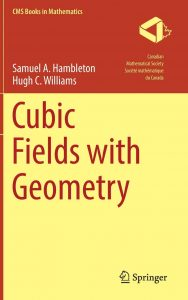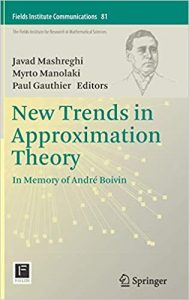# Book ReviewsBook Reviews
February 2020 (Vol. 52, No. 1)

Book Reviews bring interesting mathematical sciences and education publications drawn from across the entire spectrum of mathematics to the attention of the CMS readership. Comments, suggestions, and submissions are welcome.

Karl Dilcher, Dalhousie University (notes-reviews@cms.math.ca)

##### Cubic Fields with Geometry

S. A. Hambleton and H. C. Williams
CMS Books in Mathematics, Springer, 2018
ISBN: 978-3-030-01404-9
Reviewed by Daniel C. Mayer, Graz, AustriaThis book presents the current state of the art of working with cubic equations, polynomials and fields. It comprises a great deal of the classical knowledge and also progressive innovations by the authors.

The first two chapters exhibit foundations of algebraic number theory for the ostensive special case of cubic number fields. Irrationalities of third degree are defined as zeros of irreducible cubic polynomials, whose coefficients can be expressed as elementary symmetric polynomials, for instance trace and norm, in three conjugate zeros. The concepts of a basis of a cubic field over the rational number field and algebraic integers are introduced. With the aid of discriminants and techniques developed in the 1894 Master’s Thesis of Voronoi and by Delone/Faddeev, a classical integral basis for the maximal order is constructed initially.

As a first highlight, with crucial impact on the entire further exposition, it is shown that the unique reduced binary cubic index form of a cubic field gives rise to a particularly simple canonical unitary basis of the ring of algebraic integers over the ring of rational integers. This canonical basis admits the establishment of an elegant matrix calculus for the arithmetic in the maximal order, in particular for inverse elements and norms, similar to the Brahmagupta identity for quadratic number fields. Generally, each binary cubic form, that is, each homogeneous bivariate cubic polynomial, is linked to its quadratic Hesse covariant and its cubic Jacobi covariant by means of the Cayley syzygy, which is vital in the study of Diophantine equations. Zeros of an irreducible cubic polynomial are represented either precisely with expressions containing radicals in the formula of Cardano (inclusively goniometric formulas for the Casus Irreducibilis) or approximately by convergent sequences produced by Newton’s method.

As core structures of the arithmetic in cubic fields, the authors present the unit group, fundamental systems of units, and the regulator in logarithmic space, and on the other hand ideals, Hermite normal form, discrete lattices, and the ideal class group, connected by the analytic class number formula. The unique factorization of ideals into powers of prime ideals, inessential discriminant divisors, and the issues of the decomposition laws and contributions of rational prime numbers to discriminant and conductor are addressed thoroughly and at great length, recalling older results of Hasse of 1930 and newer investigations by Llorente/Nart of 1983.

Chapter three focusses on binary cubic forms from the view point of equivalence, reduction and composition. In this context, the Davenport/Heilbronn correspondence (1971) between classes of conjugate cubic fields and equivalent cubic forms, and the Levi parametrization (1914) of cubic orders with binary cubic forms, are covered. Reduced forms are defined with the quadratic Hesse covariant for positive discrimanants and with the quadratic Mathews form for negative discriminants. In both cases, there is a
unique reduced representative in each equivalence class.

The fourth chapter was written by Renate Scheidler. It deals with the construction of generating polynomials with small coefficients for all non-isomorphic cubic fields of an assigned fundamental discriminant. The number of these fields, whose Galois closures are unramified over a common quadratic subfield, increases exponentially with the 3-class rank of the quadratic subfield, by class field theoretic results of Hasse (1930). According to ideas of Berwick (1925) and the cubic reflection theorem by Scholz (1932), the generatig polynomials are constructed with small primitive 3-virtual units associated to the basis classes of order three in the dual quadratic resolvent field.

For negative discriminants, the infrastructure of these basis classes is used, according to an unpublished manuscript by Shanks (1987), improved by Fung (1990), and communicated here for the first time with rigorous proofs by Scheidler. For cubic fields with non-fundamental discriminants, a succinct outline of the algorithm of Belabas (1997) is given.

Chapters five and nine are devoted to several Diophantine equations viewed from Algebraic Geometry. In connection with units, the reader makes the acquaintance of the quadratic Pell equation and the group law on the associated algebraic curve over the rational integers, the cubic Pell equation of first kind with corresponding norm one torus of dimension two, in particular the norm equation for pure cubic fields, and the cubic Pell equation of second kind with associated affine variety over finite fields. Going beyond the realm of units, the cubic Pell equation of the third kind, a discriminant form equation in the sense of Győry, is discussed in connection with Pell surfaces and multiplication polynomials describing the group law.

In Chapter six, convergents of continued fraction expansions are used to express the least positive absolute values of binary quadratic and cubic forms.The greatest merit of this book is the revival of the 1896 doctoral dissertation of Voronoi, containing his generalized continued fraction algorithm for cubic orders, whose theory was interpreted geometrically by Delone and Faddeev, and is developed thoroughly in Chapters seven and eight with new proofs and original research results of the authors.

As a free module over the rational integers, an order of a cubic field can be embedded as a discrete unitary lattice into Euclidean three-space, according to the number geometric ideas of Minkowski. Voronoi defined minima of such lattices and proved that they form chains of neighboring minima on which the unit group operates and causes an orbit decomposition with finite period length, the last minimum of the first primitive period being a fundamental unit. Chapter seven exposes the properties of chains of minima, in particular the role of chains in different directions in the more intricate case of positive discriminants. Chapter eight goes into the algorithmic details and shows that it is sufficient to know how to construct the neighboring minimum of the rational unit one, which is a trivial minimum in every unitary lattice of algebraic integers. The reason is that instead of working in a fixed lattice, Voronoi switched to a similar lattice whenever a new minimum was found and started at the trivial unit one in the new unitary lattice. The authors prove a simplified new method for finding the adjacent minimum by first constructing a prepared lattice basis with distinguished properties which finally enables a selection among five possible candidates, expressed by their projections onto the two-dimensional lattice of punctures.

Two small tables of reduced index forms with covariants and an adequate collection of references complete the book. I am convinced that this book is a very valuable contribution to algebraic number theory.

Short Review

#### New Trends in Approximation TheoryIn Memory of André Boivin

Edited by J. Mashreghi, M. Manolaki, and P. Gauthier
Fields Institute Communications, Springer, 2018
ISBN: 978-1-4939-7542-6
Reviewed by Karl DilcherThis volume contains the proceedings of an international conference in memory of André Boivin (1955-2014), which took place at the Fields Institute in July of 2016. To quote from the Preface, “The impact of André Boivin’s warm personality and his fine work on Complex Approximation Theory was reflected by the mathematical excellence and the wide research range of the 37 participants. In total there were 27 talks, delivered by well-established mathematicians and young researchers. In paritcular, 19 invited lectures were delivered by leading experts of the field, from 8 different countries.”

The books begins with a summary, written by the Editors, of Boivin’s life and work, including about 20 colour photographs. The remainder of this volume consists of 14 research papers; their titles and authors are as follows:

• “A Note on the Density of Rational Functions in $A^\infty(\Omega)$“, by J. Falcón, V. Nestoridis, and I. Zadik;
• “Approximation by Entire Functions in the Construction of Order-Isomorphisms and Large Cross-Sections”, by M. R. Burke;
• “Approximation by Solutions of Elliptic Equations and Extension of  Subharmonic Functions”, by P. Gauthier and P. V. Paramonov;
• “Approximation in the Closed Unit Ball”, by J. Mashreghi, and T. Ransford;
• “A Thought on Approximation by Bi-Analytic Functions”, by D. Khavinson;
• “Chebyshev Polynomials Associated with a System of Continua”, by I. DeFrain;
• “Constrained $L^2$-Approximation by Polynomials on Subsets of the Circle”, by L. Baratchart, J. Leblond, and F. Seyfert;
• “Extremal Bounds of Teichmüller-Wittich-Belinskii Type for Planar Quasiregular Mappings”, by A. Golberg;
• “Families of Universal Taylor Series Depending on a Parameter, by E. Abakumov, J. Müller, and V. Nestoridis;
• “Interpolation by Bounded Analytic Functions and Related Questions”, by A. A. Danielyan;
• “On Two Interpolation Formulas for Complex Polynomials”, by R. Fournier and S. Ruscheweyh;
• “Operators with Simple Orbital Behavior”, by G. T. Prăjitură;
• “Taylor Series, Universality and Potential Theory”, by S. J. Gardiner; and
• “Subharmonic Images of a Convergent Sequence”, by P. Gauthier and M. Manolaki.

I had the privilege of knowing André Boivin throughout his 30-year career, cut short by his untimely death. This nicely edited and produced volume is a fitting tribute to his memory.